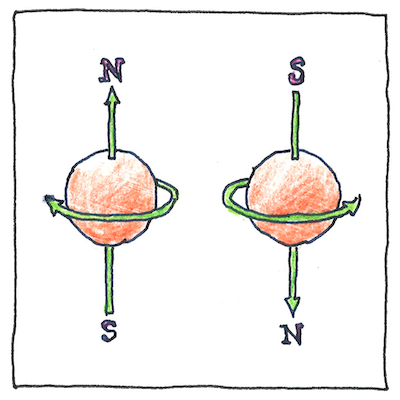# 1925,1926

## The book of science

Tom Sharp

 Wolfgang Pauli, Werner Heisenberg, Paul Dirac particle physics

## Pauli exclusion principle/Exchange interaction

• Everything doesn’t all melt together, but why not?
• The exchange interaction between two identical fermions that approach each other
• increases the expectation value of the distance between them, they say.
• Two electrons having the same spin are interdependent; however,
• they are not like two moons of a planet that would have collided long ago
• if their orbits were not sufficiently separated in space or time.
• Wolfgang Pauli, to explain patterns of spectroscopic lines
• that didn’t match the current model of the atom,
• realized that he could simplify things by assuming
• that only one electron could occupy a given state
• and that electrons were defined by four instead of three quantum numbers,
• so he introduced a fourth quantum number, the electron spin.
• We say, simply, that the four quantum numbers of two fermions
• must be different somehow.
• They must have different principal quantum numbers,
• different angular momentum quantum numbers,
• different magnetic quantum numbers,
• or different spin quantum numbers.
• This is the Pauli exclusion principle.
• Whatever electron spin is, it’s what keeps particles apart;
• out of it comes the quantization of space.

## Dimension

• We talk about quantum numbers not properties
• because these are variable values in mathematical equations.
• The first three, from Schrödinger’s wave equation,
• have physical correlatives, the size, shape, and orientation
• of electron orbitals in an atom.
• Wolfgang Pauli simply invented the fourth, the spin,
• to describe all the properties of elements in the periodic table,
• but we think of it as a kind of angular momentum
• that is not created by electrons in their orbitals.

## But why?

• We know fermions are distinct, but why?
• Why are they different in some hard-to-understand way?
• Say it’s their frequencies; no. Say it’s their “spins.”
• Say it’s that special quality that gives extent to space.
• A dancer must have space in which to dance; a knot
• that can’t be untied must have ends in opposite infinities
• or have hidden hands that clasp themselves,
• ironically afraid of intimacy with their own kind.

Maybe we should call electron spin something else, such as electron extent. The word “spin” suggests angular momentum, but it is supposed to be orthogonal to that.